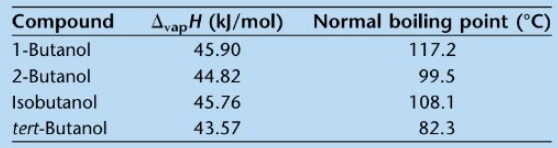Chemistry Practice Problems Clausius-Clapeyron Equation Practice Problems Solution: Four alcohols have the formula C4H9OH:1-butanol, 2...

🤓 Based on our data, we think this question is relevant for Professor McCarren's class at UIUC.

# Solution: Four alcohols have the formula C4H9OH:1-butanol, 2-butanol (or sec-butanol), isobutanol (or 2-methyl-1-propanol), and tert-butanol (or 2-methy;-2-propanol).They are examples of isomers, or compounds that have the same molecular formula but different molecular structures. The below table gives data on the isomers:Using the Clausius-Clapeyron equation, rank the isomers of butanol in order of decreasing vapor pressure at 25 °C. Does the ranking agree with any conventional wisdom based on the ΔvapH Values or the normal boiling points?

###### Problem

Four alcohols have the formula C4H9OH:

1-butanol, 2-butanol (or sec-butanol), isobutanol (or 2-methyl-1-propanol), and tert-butanol (or 2-methy;-2-propanol).

They are examples of isomers, or compounds that have the same molecular formula but different molecular structures. The below table gives data on the isomers:

Using the Clausius-Clapeyron equation, rank the isomers of butanol in order of decreasing vapor pressure at 25 °C. Does the ranking agree with any conventional wisdom based on the ΔvapH Values or the normal boiling points?Clausius-Clapeyron Equation

Clausius-Clapeyron Equation

#### Q. Ethanol has a heat of vaporization of 38.56 kJ/mol and a normal boiling point of 78.4 °C. What is the vapor pressure of ethanol at 14°C?

Solved • Mon Feb 19 2018 11:16:46 GMT-0500 (EST)

Clausius-Clapeyron Equation

#### Q. 1) The vapor pressure of dichloromethane, CH 2Cl2, at 0°C is 134 mmHg. The normal boiling point of dichloromethane is 40°C. Calculate its molar heat o...

Solved • Thu Dec 14 2017 14:33:41 GMT-0500 (EST)

Clausius-Clapeyron Equation

#### Q. Which solids will melt under applied pressure? Check all that apply.

Solved • Fri Sep 22 2017 13:14:08 GMT-0400 (EDT)

Clausius-Clapeyron Equation

#### Q. What is the vapor pressure (in atm) of a liquid at its normal boiling point? ___________________

Solved • Wed Dec 14 2016 10:38:09 GMT-0500 (EST)